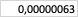# Magnetic field of a current loop

• MahalMuh

#### MahalMuh

Homework Statement
Circular current loop with radius of R = 0.3 cm and current I = 1.4 A

Calculate the magnetic field B within the distances of a) x1 = 0.17 cm and b) x2 = 2.3 cm from the axis.
Relevant Equations
B = (u0/2) * (IR^2) / (x^2 + R^2)^(3/2)
This should be pretty straightforward and my take gives B = 0.000193*T for a) and B = 0.00000063 T for b).

Or have I misunderstood something?

#### Attachments

•1612085771788.png
302 bytes · Views: 62